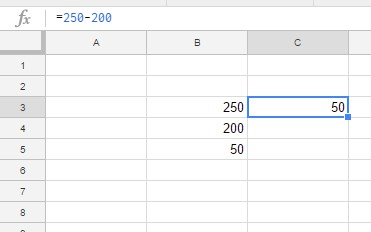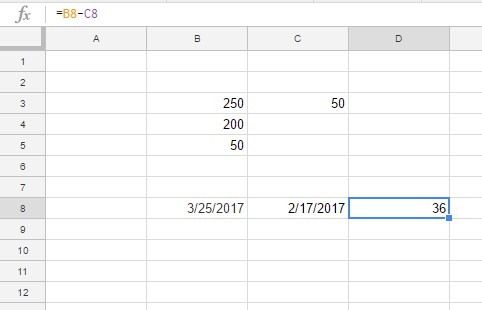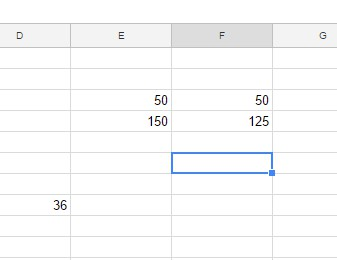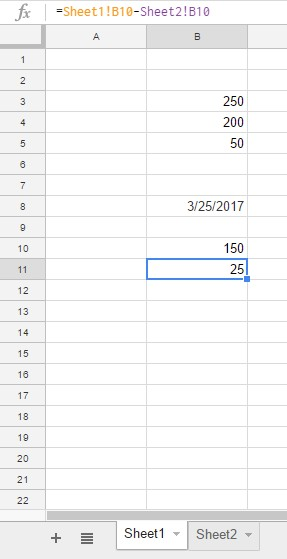# How to Subtract in Google Sheets with a FormulaSee the formula below

Since it has many of the same features as Excel, some tasks can be done in both software packages in much the same way, while others require a different approach. Regardless, if you know how to use Microsoft Excel, you’ll find Google Sheets easy to learn.

In this how-to tutorial, I will show you several different methods in Sheets for subtracting numbers from one another, a fundamental spreadsheet function.

## How to Subtract in Google Sheets

There are several methods you can use to subtract in Google Sheets. We’ll go over some of the best ones below.

Doing some basic data entry and mathematical functions are a great way to start learning a new spreadsheet software package. Some of these ways are just the same as you would do it in Excel, while others are different.

### Using the Formula bar

The simplest way to subtract two numbers in Sheets works just the same as it would in Excel — click on a cell, type what you want to subtract in the formula bar, and the cell will now contain the value of that formula.

You can use plain numbers, cell references, or both. For example, typing “=A3-200” will give you the value of cell A3 minus 200. Just like functions in Excel, in Google Sheets, you start with an equal sign.

### The MINUS Function

One notable difference between Excel and Sheets is that Google’s spreadsheet application includes a MINUS function.

The syntax for the function is: MINUS(value1, value2).

Note that MINUS is limited to subtracting just two values. Value1 is the value you want to subtract from, and value2 is the value you want to subtract from value1.To add the MINUS function to a worksheet, open a blank spreadsheet in Google Sheets. As an example, enter ‘250’ in cell B3 and ‘200’ in cell B4. Select cell B5 and enter ‘=MINUS(B3, B4)’ in the function bar. Cell B5 will return the value 50 when you press Enter.

### The Subtract Formula In Google Sheets

The MINUS function is somewhat limited, as it’s restricted to subtracting just two values. You can’t use the function to subtract numbers included in a wider range of cells, so it’s still better to subtract numbers in Google Sheets with subtraction formulas.

The subtraction formula in Google Sheets is similar to subtracting numbers with a calculator. The one difference is that you must always enter the equals (=) sign in the fx bar first and place the formula after it. You can include either actual numbers or spreadsheet cell references in the formula.

For example, select the cell C3 in a Google Sheets spreadsheet. Enter the formula ‘=250-200’ in the fx bar, then press the Enter key. 50 will appear in cell C3.Alternatively, you can subtract values entered into spreadsheet cells instead. For example, subtract the values you entered in cells B3 and B4 for the MINUS function. Select B6 and input ‘=B3-B4’ in the function bar. B6 will now include the total of B3-B4, which is otherwise 30.

Aside from integers and real numbers, you can also subtract dates with the subtraction formula. You can find the number of days between dates by entering the dates in spreadsheet cells and including their cell references in the formula. Enter the dates in the U.S. date format into Google Sheets, which is mm/dd/yyyy.

For example, select cell B8 on your Sheets spreadsheet and input ‘3/25/2017’ as the first date. Click C8 and enter ‘2/17/2017’ as the date to deduct from B8. Then select D8 to include the formula in that cell. Enter ‘=B8-C8’ in the fx bar, and press the Return key.D8 will now include the value 36, as in the shot below. As you see (and can confirm by looking at a calendar), there are 36 days between the dates.

### Subtracting Cell Range Totals in Sheets

Google Sheets users can deduct cell range totals by incorporating the SUM function within a subtraction formula. As such, you don’t need to add separate SUM functions to a couple of cells first to deduct two or more column totals.

For example, enter the values ’50’ and ‘150’ in cells E3 and E4 in your Sheets spreadsheet. Input ’50’ and ‘125’ in cells F3 and F4. Then your spreadsheet should match the example directly below.Now select F7 and click within the fx bar. Enter ‘=SUM(E4:E5)-SUM(F4:F5)’ in the function bar, and press the Return key. Cell F7 will deduct the column F total from column E, which amounts to 25.You can also include numeric values in the formula instead. Select cell F8 in the spreadsheet and enter the formula ‘=SUM(50, 150)-SUM(50, 125)’ in the fx bar. F8 will also return the same total as F7.

### Subtracting Cell Values in Multiple Worksheets

Google Sheets includes an Add Sheet button that enables you to add more worksheets to a spreadsheet. If you add multiple worksheets to your spreadsheet, you might need to subtract numbers in alternative sheets. You can do that by including sheet references within a subtraction formula.

Enter the value ‘150’ in cell B10 of Sheet 1 in your spreadsheet. Then you should press the Add Sheet button at the bottom left of Google Sheets to add Sheet 2 to the spreadsheet. Click Sheet 2 and enter ‘125’ in cell B10.Now you can add a formula to your spreadsheet that deducts B10 in Sheet 2 from B10 in the first worksheet. Select Sheet 1 and click cell B11. Enter ‘=Sheet1!B10-Sheet2!B10’ in the fx bar. B11 will include a total of 25, which is the difference between 150 and 125.

## Final Thoughts

Subtraction formulas in Sheets are flexible, so you can adjust them in various ways to deduct values as required.

Check out this Tech Junkie guide for further details on Excel subtraction formulas, many of which you can also use in Google Sheets. You might also like this article on How to get absolute value using Google Sheets.

## One thought on “How to Subtract in Google Sheets with a Formula”Mike Godshall says:
How would I apply a formula to add columns b and c then subtract column d generating a total in column E

Disclaimer: Some pages on this site may include an affiliate link. This does not effect our editorial in any way.

Todays Highlights